# Non-smoothable manifold

(diff) ← Older revision | Latest revision (diff) | Newer revision → (diff)

A piecewise-linear or topological manifold that does not admit a smooth structure.

A smoothing of a piecewise-linear manifoldis a piecewise-linear isomorphism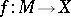, whereis a smooth manifold. Manifolds that do not admit smoothings are said to be non-smoothable. With certain modifications this is also applicable to topological manifolds.

Example of a non-smoothable manifold. Let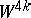,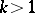, be a-dimensional Milnor manifold (see Dendritic manifold). In particular,is parallelizable, its signature is 8, and its boundaryis homotopy equivalent to the sphere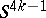. Glueing toa cone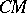over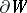leads to the space. Sinceis a piecewise-linear sphere (see generalized Poincaré conjecture),is a piecewise-linear disc, so thatis a piecewise-linear manifold. On the other hand,is non-smoothable, since its signature is 8, while that of an almost-parallelizable (that is, parallelizable after removing a point)-dimensional manifold is a multiple of a numberthat grows exponentially with. The manifoldis not diffeomorphic to the sphere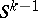, that is,is a Milnor sphere.

A criterion for a piecewise-linear manifold to be smoothable is as follows. Let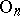be the orthogonal group and let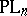be the group of piecewise-linear homeomorphisms ofpreserving the origin (see Piecewise-linear topology). The inclusion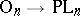induces a fibration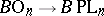, whereis the classifying space of a group. Asthere results a fibration, the fibre of which is denoted by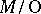. A piecewise-linear manifoldhas a linear stable normal bundlewith classifying mapping. Ifis smoothable (or smooth), then it has a stable normal bundle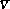with classifying mappingand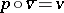. This condition is also sufficient, that is, a closed piecewise-linear manifoldis smoothable if and only if its piecewise-linear stable normal bundle admits a vector reduction, that is, if the mappingcan be "lifted" to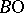(there is a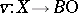such that).

Two smoothingsand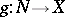are said to be equivalent if there is a diffeomorphism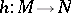such thatis piecewise differentiably isotopic to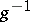(see Structure on a manifold). The setsof equivalence classes of smoothings are in a natural one-to-one correspondence with the fibre-wise homotopy classes of liftingsof. In other words, whenis smoothable,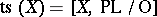.

How to Cite This Entry:
Non-smoothable manifold. Encyclopedia of Mathematics. URL: http://encyclopediaofmath.org/index.php?title=Non-smoothable_manifold&oldid=17941
This article was adapted from an original article by Yu.I. Rudyak (originator), which appeared in Encyclopedia of Mathematics - ISBN 1402006098. See original article